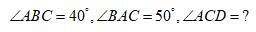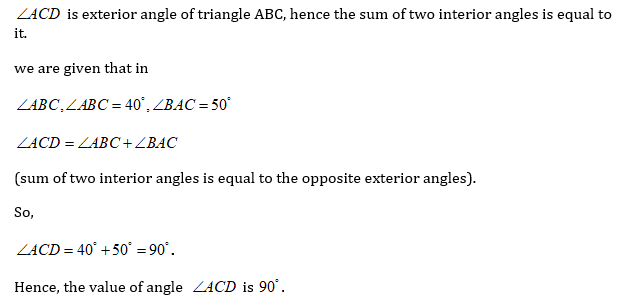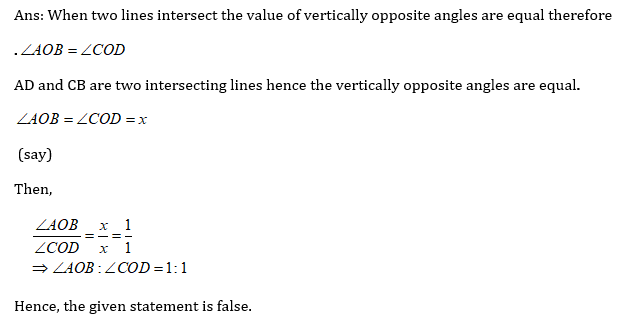×## Pair of Lines - 88tuition

#### Mathematics

An Introduction to Pairs of Lines

We use a pair of lines to study the properties of lights in science. For example in reflection or refraction when light reflects or passes from one medium to another we make use of relations between parallel and intersecting lines. On the chess board, we use a beautiful combination of parallel and intersecting lines. Lines are the most fundamental entity in geometry. Most of the shapes that we observe in real life are generally made of different combinations and orientations of lines in some special order.

## Points, Lines, and line segment

• A point is a precise position that is symbolized by a little dot formed with a fine pen on a piece of paper.

• A line is defined as a configuration of points that has length but neither breadth nor height.

• A line segment is a line made up by joining at least two points. A line has no endpoints but line segments have two endpoints. Lines with one endpoint are known as Ray.

• In the following figure, points, lines, line segments, and rays are demonstrated:Figure: (a) Point, (b) Line, (c) Line segment, and (d) Ray

## Pairs of line

Pairs of lines are those two lines which are somehow related to each other. These relations can be parallel lines, perpendicular lines, intersections, or transversal lines.

## A pair of parallel lines

A line is said to be parallel to another line if both of them have the same slope or simply if pairs of lines are parallel when they do not have any intersection.Figure: A pair of parallel lines

Lines x and y are parallel in the illustration above, thus we'll say that line x is parallel to line y or vice versa. We are aware that two lines in the same plane are either parallel to one another or intersect at some point. Regardless of how far apart they are, these two parallel lines, x, and y, resting in the same plane, are parallel if they never intersect.

Parallel lines are represented by symbols ∥ . We can write that x∥y  means x and y are parallel to each other.

## Intersecting lines

Any two lines, line segments, or rays that share a common point are said to be intersecting lines. The point where they intersect is known as the point of intersection. When two lines intersect they form an angle at the point of intersectionFigure: Intersection line and O is the point of intersection

### Perpendicular lines

A line is said to be perpendicular to another line if the angle between the two lines is 90 degrees. In other words, two lines are perpendicular to one another if they connect at the right angle. Take a look at the figure below. Since the lines AB and CD cross at right angles, we can say that they are perpendicular to each other. They are represented by the symbol ‘‘. Symbolically we can write AB CD which means AB is perpendicular to CD or vice versa.Figure: Perpendicular lines

## Transversal

A transversal line is a straight line that intersects two or more other straight lines at different places. Roads can be built with intersections, such as intersecting streets, to allow for easy traffic movement from many directions and reduce congestion. When a third street intersects two or more parallel streets, a transversal will form. In the diagram, l and m are parallel lines, and t is a transversal line. Angles are created when two lines come together at a point, as we already know from intersecting lines. Similar to this, various angles are created at each location where a transverse line intersects two or more lines.Figure: Transversal

## Properties of transversal

• Vertically opposite angles are equal.

• Corresponding angles are equal.

• The interior angles formed on the same side of the transversal are supplementary.

• Alternate angles are equal.Figure: Transversal line (1,2,3,4,5,6,7,8 are symbols used for angles)

### Interesting facts

• Distance between two parallel lines is always constant.

• Two parallel lines are always coplanar.

• At the intersection point, opposite angles are equal to each other.

• If two lines are parallel then their slope must be equal.

## Solved problems

1. Nick draws a triangle as shown in the figure and asks Tom to solve it, where he says that, help Tom in solving the question.2. If Mr. Jack draws two intersecting lines and says that the ratio of these two lines is  4:3. Check whether the statement is true or false?1. What does a straight line mean?

The geometry object known as a line stretches on both edges and is defined as an object with zero width. Simply put, a straight line is an uncurved path. A straight line is one that has no curves and stretches to infinity on both edges.

1.  If two lines are intersecting each other, is it possible that they have more than one point of intersection?

No it is not possible. Two lines can have a maximum single point of intersection.

1. How can a line's perpendicularity be determined?

A pair of non-vertical lines in the same plane are said to be perpendicular if they cross at a right angle. Vertical and horizontal lines, or the planes of the coordinate plane, are opposite to one another.

#### Recent Posts